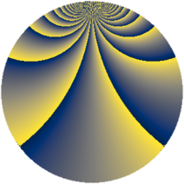# Properties

 Label 784.4.btLevel $784$ Weight $4$ Character orbit 784.bt Rep. character $\chi_{784}(37,\cdot)$ Character field $\Q(\zeta_{84})$ Dimension $8016$ Sturm bound $448$

# Related objects

## Defining parameters

 Level: $$N$$ $$=$$ $$784 = 2^{4} \cdot 7^{2}$$ Weight: $$k$$ $$=$$ $$4$$ Character orbit: $$[\chi]$$ $$=$$ 784.bt (of order $$84$$ and degree $$24$$) Character conductor: $$\operatorname{cond}(\chi)$$ $$=$$ $$784$$ Character field: $$\Q(\zeta_{84})$$ Sturm bound: $$448$$

## Dimensions

The following table gives the dimensions of various subspaces of $$M_{4}(784, [\chi])$$.

Total New Old
Modular forms 8112 8112 0
Cusp forms 8016 8016 0
Eisenstein series 96 96 0

## Trace form

 $$8016q - 26q^{2} - 26q^{3} - 26q^{4} - 26q^{5} - 20q^{6} - 104q^{8} + O(q^{10})$$ $$8016q - 26q^{2} - 26q^{3} - 26q^{4} - 26q^{5} - 20q^{6} - 104q^{8} - 20q^{10} - 26q^{11} - 26q^{12} - 20q^{13} - 216q^{14} - 40q^{15} - 26q^{16} - 52q^{17} - 50q^{18} - 12q^{19} - 100q^{20} + 30q^{21} - 524q^{22} - 526q^{24} - 26q^{26} + 88q^{27} + 510q^{28} - 20q^{29} + 4q^{30} - 4488q^{31} - 516q^{32} - 52q^{33} + 668q^{34} + 454q^{35} + 236q^{36} - 26q^{37} - 246q^{38} + 1474q^{40} - 1998q^{42} - 20q^{43} - 5724q^{44} + 528q^{45} - 344q^{46} - 1932q^{47} + 928q^{48} - 48q^{49} - 1444q^{50} + 566q^{51} + 1576q^{52} - 26q^{53} + 1246q^{54} + 3144q^{56} + 1668q^{58} - 714q^{59} + 7942q^{60} - 26q^{61} + 1832q^{62} + 1324q^{63} + 1072q^{64} - 52q^{65} - 10226q^{66} - 936q^{67} - 1278q^{68} + 88q^{69} + 2124q^{70} - 1050q^{72} - 1104q^{74} + 332q^{75} + 7630q^{76} + 662q^{77} + 2336q^{78} - 24q^{79} - 8900q^{80} - 51568q^{81} + 13878q^{82} - 2460q^{83} + 22508q^{84} - 520q^{85} - 2840q^{86} + 1976q^{88} + 9304q^{90} - 11348q^{91} - 660q^{92} + 244q^{93} + 7682q^{94} - 3916q^{95} + 53004q^{96} - 96q^{97} + 13634q^{98} + 2760q^{99} + O(q^{100})$$

## Decomposition of $$S_{4}^{\mathrm{new}}(784, [\chi])$$ into newform subspaces

The newforms in this space have not yet been added to the LMFDB.